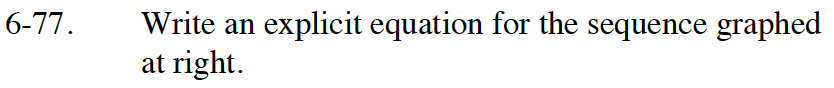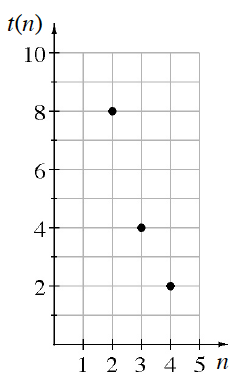Home > GB8I > Chapter cc46 > Lesson cc46.2.3 > Problem6-77

6-77.

Write an explicit equation for the sequence graphed at right. Homework Help ✎$\text{The multiplier for this graph is \frac{1}{2}.}$

Work backward to find term 0, or the starting value.

Use the starting value and the multiplier to write the equation.

$t(n)=32\left( \frac{1}{2} \right)^n$